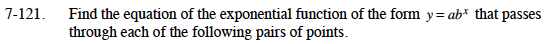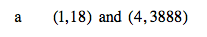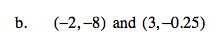Home > CCA2 > Chapter 7 > Lesson 7.2.1 > Problem7-121

7-121.
1. Find the equation of the exponential function of the form y = abx that passes through each of the following pairs of points. Homework Help ✎

1. (1, 18) and (4, 3888)

2. (–2, –8) and (3, –0.25)Construct two equations by substituting the coordinates
for x and y.

18 = ab1
3888 = ab4

Use substitution to find the value of a and b.

$a = \frac{18}{b}$

$3888 = \frac{18}{b}b^4$

y = 3(6)xUse the same method as part (a).